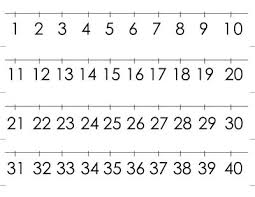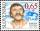# The product 3

The product of two numbers is 15 ⁵/₆. If one of the numbers is 5 ²/₃, find the other.

b =  2.7941

### Step-by-step explanation:Did you find an error or inaccuracy? Feel free to write us. Thank you!## Related math problems and questions:

• The product 2The product of two functions is 10. If one of them is 2 1/3, find the other one.
• Two numbers and its productThe product of two numbers are 2/3. If on of them is 1/10, what is the other?
• The sum 12The sum of 3 mixed numbers is 20 13/15. two of the numbers are 6 1/3 and 7 5/6. what is the third number?
• To improper fractionChange mixed number to improper fraction a) 1 2/15 b) -2 15/17
• Land costThe land is worth 33295 CZK. 1st owner has 5/15, 2nd owner has 4/15, 3rd owner has 1/5 4th owner has 1/5. How much CZK each of them will receive?
• Sum of 18Sum of two fractions is 4 3/7. If one of the fractions is 2 1/5 find the other one .
• Evaluate fractionsThe difference  of 5 1/2 and 2/3 is added to the product of 5/6 and 1/2
• Find unknown 2Find unknown denominator: 2/3 -5/? = 1/4
• The length 5The length and breadth of rectangular field is 3 1/5 m  and 1 3/7 m. Find the area of rectangular field.
• Fractions and mixed numerals(a) Convert the following mixed numbers to improper fractions. i. 3 5/8 ii. 7 7/6 (b) Convert the following improper fraction to a mixed number. i. 13/4 ii. 78/5 (c) Simplify these fractions to their lowest terms. i. 36/42 ii. 27/45 2. evaluate the follow
• Product of two fractionsProduct of two fractions is 9 3/5 . If one of the fraction is 9 3/7. Find the other fraction.
• Arithmetic progression 2The 3rd term of an Arithmetic progression is ten more than the first term, while the fifth term is 15 more than the second term. Find the sum of the 8th and 15th terms of the Arithmetic progression if the 7th term is seven times the first term.
• Simplify expression with mixedWhen (7¹/3 + 2¾)÷(2 + 2¼ × 1¹/3) is simplified the result is?
• Quotient and productIf the quotient of [8/5 divided by 8/10] is added to the product of [8/14 x 7/12 x 3/8], what is the sum?
• The length 2The length of a rectangle is 12y2 – 15y + 8 and the width is 7y – 11. Find the area of the rectangle
• Two numbers 11The sum of two rational numbers is (-2). If one of them is 3/5, find the other.
• Sum of mixedThe sum of two mixed numbers is 15½. One of the number is 5 ¾, what is the other?